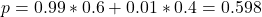## In Exercise 6, suppose that there is a probability of .01 that a digit is incorrectly sent over a communication channel (i.e., that a digit

Question

In Exercise 6, suppose that there is a probability of .01 that a digit is incorrectly sent over a communication channel (i.e., that a digit sent as a 1 is received as a 0, or a digit sent as a 0 is received as a 1). Consider a message that consists of exactly 60% 1s. a. What is the proportion of 1s received at the end of the channel

in progress 0
6 months 2021-07-17T12:30:05+00:00 1 Answers 6 views 0

The proportion of 1s received at the end of the channel is of 0.598.

Step-by-step explanation:

In this question:

60% of the bits sent are 1, 100 – 60 = 40% of the bits sent are 0.

For each bit receive, 0.01 probability of an error, so 0.99 probability it is correct.

a. What is the proportion of 1s received at the end of the channel?

99% of 60%(sent 1s, received 1).

1% of 40%(sent 0s, received 1). SoThe proportion of 1s received at the end of the channel is of 0.598.